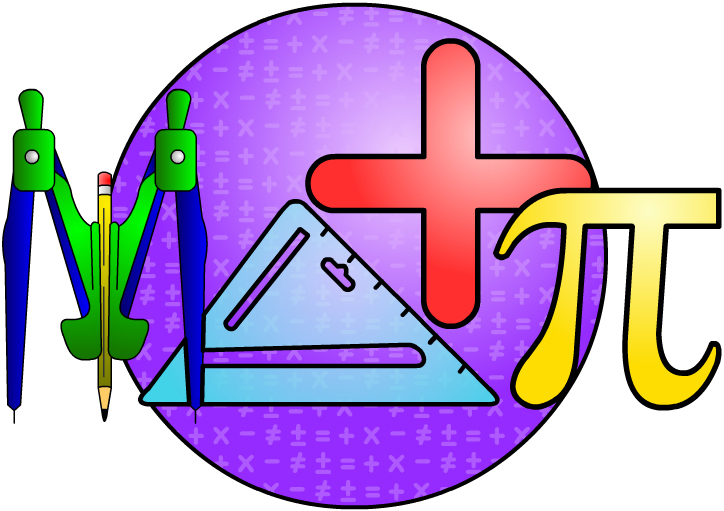# Math###### MIDDLE SCHOOL MATHEMATICS

The study of mathematics at Brandon Academy comprises four key components: mastery of traditional mathematical concepts that prepare all students for success in future mathematics courses, effectively communicating mathematics both in written and oral form, building creativity and confidence in problem-solving, and providing challenging opportunities for individual growth through competition and classroom environments. Students are assessed annually by the mathematics faculty to determine appropriate mathematics placement.

The goal of sixth grade mathematics is for all students to develop the critical thinking skills and concepts that are the building blocks for future mathematics courses. Sixth grade students continue to practice and master operations on expressions involving real numbers. As understanding improves, there is an improvement in the ability to recognize and convert numbers to equivalent quantities based on the needs of applications. Students continue to expand their study of algebra, statistics, geometry, measurement and probability.

Skills to be mastered include: The Real Number System (including fractions, decimals, integers, whole and irrational numbers); Measurement; Number Theory; Scientific Notation; Equations, inequalities and algebraic thinking; Ratios, rates, proportions and percent; Geometry (both two- and three-dimensions); Graphing on the coordinate plane; Displaying and analyzing data; Probability; Mental math; Test-taking strategies.

The goal of seventh grade mathematics is to solidify foundations in algebra, geometry, statistics, measurement and probability in preparation for the transition to a formal study of algebra in the eighth grade. Word problem applications will be used throughout the course to develop independence and confidence when given problem-solving situations that have not been previously or explicitly taught.

Skills to be mastered include: Displaying and analyzing data; Defining and using variables to write algebraic expressions that represent real-world situations; Solving equations and inequalities; Using formulas to measure two- and three-dimensional shapes; Graphing linear functions; Calculate basic probabilities with set and geometric applications; Perform basic polynomial operations; Applying integer concepts and operations; Applying rational number concepts and operations; Defining and applying proportional relationships in problem-solving.

Pre-Algebra

The goal of Pre-Algebra is to extend, enhance and expand the study of mathematics from the sixth and seventh grades in anticipation of the formal study of algebra. Students will work to master and retain pre-algebra concepts while working on introductory algebra skills. The focus is on building mathematical maturity through analysis and application of skills when encountering problem-solving opportunities.

Skills to be mastered include: Identifying appropriate units of measure and conversions; Continuing to build number sense; Applying integer concepts and operations; Analyzing data and statistics; Finding probability of independent and dependent events; Applying formulas to measure two-and three-dimensional geometric shapes; Using proportional thinking with percent applications; Identifying patterns in algebraic transformations through solving equations and inequalities.

Algebra I

The goal of the Algebra I course is to give each student a solid foundation in algebraic thinking and methodology in conjunction with experience and fluency in problem-solving applications. This is a critical foundation course essential for future success in high school mathematics.

Skills to be mastered include: Identifying properties of numbers; Mastering operational fluency with all types of real numbers; Applying accurate and efficient transformations to solve multi-step equations and inequalities; Graphing linear and quadratic functions and inequalities; Applying and transforming formulas; Writing and solving systems of equations and inequalities; Simplifying and performing operations with exponents; Solving radical expressions and solving radical equations; Factoring and performing operations on polynomial expressions; Writing and solving proportional equations; Simplifying rational expressions and solving rational equations; Using algebraic skills and techniques to solve problems involving applications of all topics.